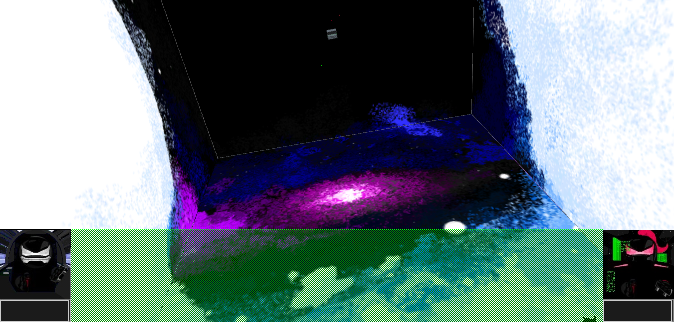[Games]

"Version 4.1.2 Jade Red and Cannonballhead XYZ Axis Coordinates Distance Calculator Calculator Version 4.1.2 Calculator"

# Enter the coordinates  then proceed to step #1

1st Coordinate = (x, y, z) =
(, , )

2nd Coordinate = (x, y, z) =
(, , )

# Step #1

(The coordinates you entered above are now shown in the equation here in step #1)

# Click each of the red, green, and blue subtraction equation buttons to show an answer and a color. Then place each answer into the matching color boxes of step #2.

distance = √(( - )2 + ( - )2 + ( - )2)

(x₁ - x₂)=

=
+
(y₁ - y₂)=

=
+
(z₁ - z₂)=

= )

# Step #3

After you have answers in each lime colored box of step #2 you can press this button (place this orange color answer into the orange and purple boxes of step 4)

# Step #4

Distance =
√()
(Orange Box = This is your answer written as a square root. You will need to simplify square root answers when necessary) [example: √12 is the same as 2√3. √6 is not the same as 2√3.]
√() (Click this button to use the √(square root) function)## Elevation Angles

Examples to use:

• Use the distance you found in the 'XYZ Axis Coordinates Distance Calculator Calculator' as the hypotenuse length.
• A positive form of the green colored answer in step #1 of the XYZ coordinates calculator can be used as the measurement of the distance of the other player's height above or below you. It is a y-axis length and is the axis your base's drone will travel on, in a vertical direction. (If the green answer in step #1 is zero there is no elevation angle to solve for.)
• Insert 2 lengths into a set of yellow and turquoise bordered boxes. Then press the red 'PUSH' button.
• Copy and paste answers into the matching color input boxes. Use the corresponding buttons to provide answers into the next color box.

#### If you know the length opposite the angle and the hypotenuse, use this calculator.

/
(note: Hypotenuse must be longer than the opposite length)

Degrees

#### If you know the adjacent length and the hypotenuse length, use this calculator.

/
(note: Hypotenuse must be longer than the adjacent length)

Degrees

#### If you know the length opposite the angle and the adjacent length, use this calculator.

/

Degrees

``` Arc Length = 2πr(angle° ÷ 360) Surface Area of a Sphere = 4πr2 Volume of a Sphere = (4/3)πr3 Density = mass/volume Circumference of a Circle C = 2πr    =    C = πd The equation of a circle with center (a, b), radius (r), and outer coordinate (x, y) : (x - a)2 + (y - b)2 = r2 The equation of a sphere with center (a, b, c), radius (r), and a coordinate located a distance equal to the radius from center represented as (x, y, z) : ((x - a)2 + (y - b)2 + (z - c)2) = r2 Pythagorean Theorem a2 + b2 = c2 ```

X minimum -206265 and maximum 206265
Y minimum -206265 and maximum 206265
Z minimum -206265 and maximum 206265

milliseconds
(The digital time display is stopped time in 24 hour format; hours:minutes:seconds)

X Y Z
Computer
Computer
Coins Red Orange Yellow Green Blue Indigo Violet
0 0 0 0
```60 red      = 1 Coin  //occurs every hour
60 orange   = 1 red  //occurs every minute
1000 yellow = 1 orange  //occurs every second
10 green    = 1 yellow  //occurs every .001 of a second
10 blue     = 1 green  //occurs every .0001 of a second
10 indigo   = 1 blue  //occurs every .00001 of a second
10 violet   = 1 indigo  //occurs every .000001 of a second

```

Movement Simulator version 1.1.1

X

Y

Z

To calculate the speed required, to travel a known distance and time, use this equation:
Distance/Time = Rate

÷ = Rate

To calculate the amount of time, to travel a known distance and speed, use this equation:
Distance/Rate=Time

÷ = Time

To calculate the distance, of a known speed and time, use this equation:
Distance = Rate × Time

× = DistancePASS CODE LOCK SIMULATOR

# Pirates Dice

• Five of a kind
• Four of a kind
• Full House
• Straight
• Three of a kind
• Two Pair
• One Pair
• High Dice

# Sticky Notes